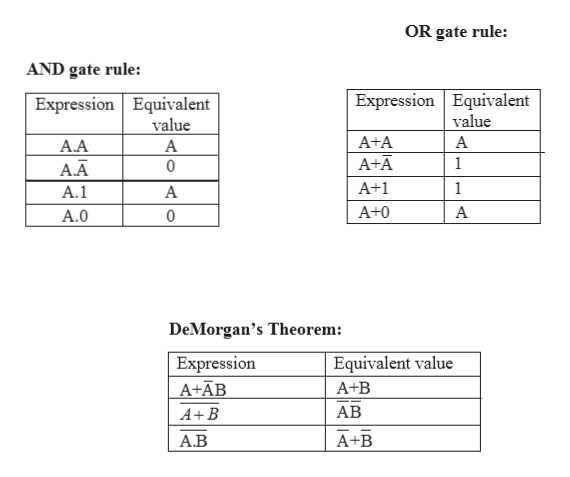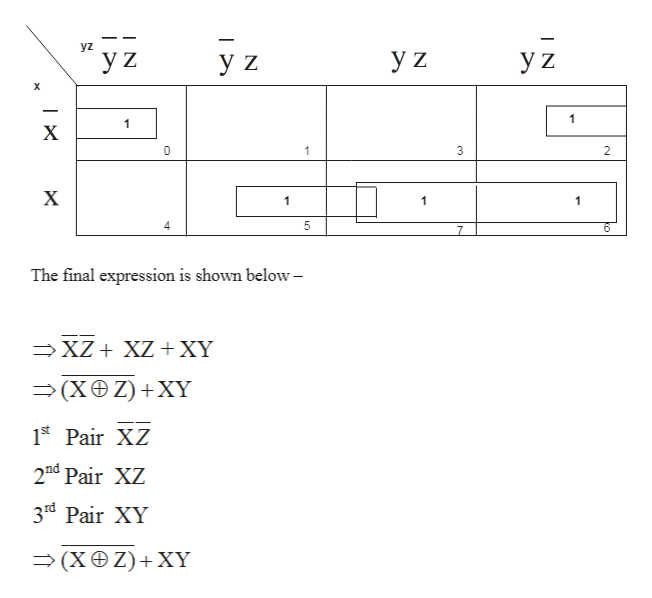# Please explain, as simplistically (but thoroughly) as possible, how to develop a k-map from a truth table to be used to simplify an equation.Examples:xz + (xy + ~z)~xyz + yz + x~y

Question
110 views

Please explain, as simplistically (but thoroughly) as possible, how to develop a k-map from a truth table to be used to simplify an equation.

Examples:

1. xz + (xy + ~z)
2. ~xyz + yz + x~y
check_circle

Step 1

Rules to be followed to simplify the Boolean expression are given below-help_outlineImage TranscriptioncloseOR gate rule: AND gate rule: Expression Equivalent Expression Equivalent value value A+A A A.A А A+A 1 А.А 0 A+1 1 А.1 A+0 A A.O 0 DeMorgan's Theorem Expression A+ĀB Equivalent value A+B АВ A+B А.В A+B fullscreen
Step 2

Simplify the Boolean equations: xz + (xy + ~z)

For simplifying the Boolean expression, use Karnaugh Map and follow the below steps-

1. a) Fill 1 at decimal equivalent number 0,2,5,6,7 as per the given question accordingly in the K-map.
2. b) Then pairing will be done in the K-map.

c)There will be three pairs formed in this K -map as shown below –help_outlineImage Transcriptioncloseyz УZ Уz X 1 1 X 0 3 X 1 1 4 5 The final expression is shown below- XZXZ+XY (X Z) XY 1S Pair XZ 2nd Pair XZ 3rd Pair XY — (Х®Z)+XY IN I N fullscreen
Step 3

Now, to find the truth table for the final expression xz + (xy + ~z), firstly calculate the truth tabl...

### Want to see the full answer?

See Solution

#### Want to see this answer and more?

Solutions are written by subject experts who are available 24/7. Questions are typically answered within 1 hour.*

See Solution
*Response times may vary by subject and question.
Tagged in

### Computer Science